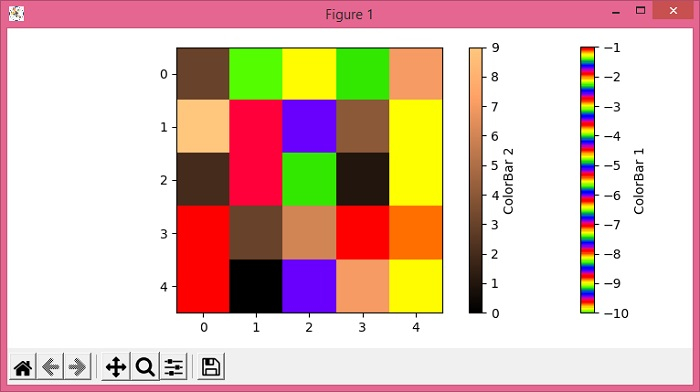# How to show two different colored colormaps in the same imshow Matplotlib?

To show two different colored colormaps in the same imshow matplotlib, we can take the following steps −

• Set the figure size and adjust the padding between and around the subplots.

• Make a 2D matrix of 5×5 dimension.

• Get masked matrix, data1 and data2, with positive and negative values.

• Create a figure and a set of subplots.

• Display the data as an image, i.e., on a 2D regular raster, with data1 and data2.

• To make two different colorbars, use colorbar method.

• Set the colorbar for both the images.

• Set the label of the colorbars.

• To display the figure, use show() method.

## Example

import matplotlib.pyplot as plt
import numpy as np

plt.rcParams["figure.figsize"] = [7.50, 3.50]
plt.rcParams["figure.autolayout"] = True

img = np.random.randint(-10, 10, (5, 5))

data1 = np.ma.masked_array(img, img >= 0)
data2 = np.ma.masked_array(img, img < 0)

fig, ax = plt.subplots()

img1 = ax.imshow(data1, cmap="prism_r")
img2 = ax.imshow(data2, cmap="copper")

bar1 = plt.colorbar(img1)
bar2 = plt.colorbar(img2)

bar1.set_label('ColorBar 1')
bar2.set_label('ColorBar 2')

plt.show()

## Output